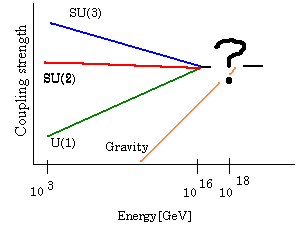# SUPERSTRINGS! Why Strings?

While the Standard Model has been very successful in describing most of the phenomemon that we can experimentally investigate with the current generation of particle acceleraters, it leaves many unanswered questions about the fundamental nature of the universe. The goal of modern theoretical physics has been to find a "unified" description of the universe. This has historically been a very fruitful approach. For example Einstein's theory of Special Relativity unifies the forces of electricity and magnetism into the electromagnetic force. The Nobel prize winning work of Glashow, Salam, and Weinberg successfully showed that the electromagnetic and weak forces can be unified into a single electroweak force. There is actually some pretty strong evidence that the forces of the Standard Model should all unify as well. When we examine how the relative strengths of the strong force and electroweak force behave as we go to higher and higher energies, we find that they become the same at an energy of about 10^16 GeV. In addition the gravitational force should become equally important at an energy of about 10^19 GeV.The goal of string theory is to explain the "?" in the above diagram.

The characteristic energy scale for quantum gravity is called the Planck Mass, and is given in terms of Planck constant, the speed of light, and Newton's constant,It seems that in its final form string theory will be able to provide answers to answer questions like:

• Where do the four forces that we see come from?
• Why do we see the various types of particles that we do?
• Why do particles have the masses and charges that we see?
• Why do we live in 4 spacetime dimensions?
• What is the nature of spacetime and gravity?